# 3D Printing: MSLA printing Triply Periodic Minimal Surfaces (TPMS) – Gallery

• 2023/07/25: adding 20mm/40mm TPMS photos
• 2023/03/12: starting write-up, and published

20mm cubes of several Triply Periodic Minimal Surfaces (TPMS) as explored at Generative Parametric Infill Geometries printed with MSLA (Anycubic Photon Mono 4K) at 35 μm XY, 50 μm Z:

Most of the cubes were printed without support, the cylindrical and spherical projections required supports.

## Closeups

20mm and 40mm cubes of TPMS mounted on canvases:

# 3D Modeling: Elegant Pieces in OpenSCAD with rcube(), rcylinder() and chainhull()

• 2020/12/31: `rcube()` extended, `RCUBE_FLAT{BOTTOM, TOP, FRONT, BACK, LEFT, RIGHT}` support added, `rcylinder()` with `RCYLINDER_FLAT{TOP, BOTTOM}`
• 2020/12/30: `rcube()` source code extended, support `RCUBE_FLATX, RCUBE_FLATY, RCUBE_FLATZ`
• 2020/12/28: inital post

While working on Ashtar D (Classic XY) I looked at some pieces I rushed to design with `cube()` and `hull()` and they didn’t appeal to me – yes, it kind of hurt my eyes.

A while back I coded a simple `rcube([x,y,z],r)` which takes `r` as a radius for the edges, internally it’s an OpenSCAD module which uses 8 spheres and hulls them together, providing round edges; but I hesitated to actually use it in my designs – until now. Further I thought, let’s do the same with `cylinder()` using `rcylinder(d=10,h=5,r=1)` providing round edges by using two torii and hull them together.

These two new functions, `rcube([x,y,z],r)` and `rcylinder(h,d,r)` allow to create more organic and elegant pieces, see for yourself:

## From Bulky To Elegance

The position of the Y pulley mount is given, a bit of an X- & Y-offset to ensure printable area is not sacrificed for the Y carriage:

## Using Chained Hulls

And another example . . . replacing `hull()` with `chainhull()`:

The final version is composed by only 3 pieces chain hulled together:

```difference() {
chainhull() {
rcylinder(...);
translate([0,0,-20]) rcube(...);
translate([...,-60]) rcube([5,20,50],2); // 2020 mount plate
}
rcube(...);     // pulley cutout
}```

## rcube() & rcylinder()

```rcube();
translate([5,0,0]) rcube(0.75);
translate([10,0,0]) rcube([2,1,1],0.2);

translate([0,2,0]) rcube([2,1,1],0.2,false);
translate([5,2,0]) rcube([2,1,1],0.2,true);

translate([0,4,0]) rcube([2,1,1],0.2,RCUBE_FLATX);
translate([5,4,0]) rcube([2,1,1],0.2,RCUBE_FLATY);
translate([10,4,0]) rcube([2,1,1],0.2,RCUBE_FLATZ);

translate([0,6,0]) rcube([2,1,1],0.2,RCUBE_FLATBOTTOM);
translate([5,6,0]) rcube([2,1,1],0.2,RCUBE_FLATTOP);

translate([0,8,0]) rcube([2,1,1],0.2,RCUBE_FLATFRONT);
translate([5,8,0]) rcube([2,1,1],0.2,RCUBE_FLATBACK);

translate([0,10,0]) rcube([2,1,1],0.2,RCUBE_FLATLEFT);
translate([5,10,0]) rcube([2,1,1],0.2,RCUBE_FLATRIGHT);

translate([0+1,14,0]) rcylinder(3,1.5,0.2);
translate([3+1,14,0]) rcylinder(3,1.5,0.2,false);
translate([6+1,14,0]) rcylinder(3,1.5,0.2,RCYLINDER_FLATBOTTOM);
translate([9+1,14,0]) rcylinder(3,1.5,0.2,RCYLINDER_FLATTOP);```

The library code (I might later release it as a separate library):

```// Title: rcube(), rcylinder() & torus()
// Author: Rene K. Mueller
// Version: 0.0.2

RCUBE_FLATX = [false,true,true];
RCUBE_FLATY = [true,false,true];
RCUBE_FLATZ = [true,true,false];
RCUBE_FLATBOTTOM = [false,false,false,false,true,true,true,true];
RCUBE_FLATTOP = [true,true,true,true,false,false,false,false];
RCUBE_FLATFRONT = [false,false,true,true,false,false,true,true];
RCUBE_FLATBACK = [true,true,false,false,true,true,false,false];
RCUBE_FLATLEFT = [false,true,true,false,false,true,true,false];
RCUBE_FLATRIGHT = [true,false,false,true,true,false,false,true];

module rcube(a=1,r=0.1,rd=[true,true,true],center=false,\$fn=32) {
if(FAST_RCUBE)
cube(a);
else {
x = len(a) ? a : a;
y = len(a) ? a : a;
z = len(a) ? a : a;
rd = len(rd) ? rd : [rd,rd,rd];

if((len(rd)==3 && rd && rd && rd) || (len(a)==0 && rd)) // rd=[true,true,true] or true
hull() {
translate([r,r,r]) sphere(r);
translate([x-r,r,r]) sphere(r);
translate([x-r,y-r,r]) sphere(r);
translate([r,y-r,r]) sphere(r);
translate([r,r,z-r]) sphere(r);
translate([x-r,r,z-r]) sphere(r);
translate([x-r,y-r,z-r]) sphere(r);
translate([r,y-r,z-r]) sphere(r);
}
else                                                        // anything else
hull() {
translate([r,r,r]) rcube_prim(r,rd,0);
translate([x-r,r,r]) rcube_prim(r,rd,1);
translate([x-r,y-r,r]) rcube_prim(r,rd,2);
translate([r,y-r,r]) rcube_prim(r,rd,3);
translate([r,r,z-r]) rcube_prim(r,rd,4);
translate([x-r,r,z-r]) rcube_prim(r,rd,5);
translate([x-r,y-r,z-r]) rcube_prim(r,rd,6);
translate([r,y-r,z-r]) rcube_prim(r,rd,7);
}
}
}

module rcube_prim(r,rd,i) {
a = len(rd);
if(a<=3) {
if(a && rd && rd && rd)
sphere(r);
else if(a && rd && rd)
translate([0,0,-r]) cylinder(r=r,h=r*2);
else if(a && rd && rd)
translate([-r,0,0]) rotate([0,90,0]) cylinder(r=r,h=r*2);
else if(a && rd && rd)
translate([0,-r,0]) rotate([-90,0,0]) cylinder(r=r,h=r*2);
else
translate([-r,-r,-r]) cube(r*2);
} else
if(rd[i])
sphere(r);
else
translate([-r,-r,-r]) cube(r*2);
}

RCYLINDER_FLATBOTTOM = [false,true];
RCYLINDER_FLATTOP = [true,false];

module rcylinder(h=2,d=1,r=0.1,rd=[true,true],\$fn=40) {
if(FAST_RCYLINDER)
cylinder(d=d,h=h);
else
hull() {
translate([0,0,r])
if(len(rd) && rd) torus(do=d,di=r*2); else translate([0,0,-r]) cylinder(d=d,h=r);
translate([0,0,h-r])
if(len(rd) && rd) torus(do=d,di=r*2); else cylinder(d=d,h=r);
}
}

module torus(do=2,di=0.1,a=360) {
rotate_extrude(convexity=10,angle=a) {
translate([do/2-di/2,0,0]) circle(d=di,\$fn=20);
}
}```

## chainhull()

```module chainhull() {
for(i=[0:1:\$children-2])
hull() {
children(i);
children(i+1);
}
}```

There is one drawback using `chainhull() { }` as you can’t use conditional `if else` with `{ }` within as it combines them as a group and becomes a child structure and so it will act as `hull()`, so you only can list non-conditional pieces within `chainhull()` as of OpenSCAD 2019.05, perhaps at a later time this limit vanishes.

That’s it.

# 3D Printing: Random Prints #1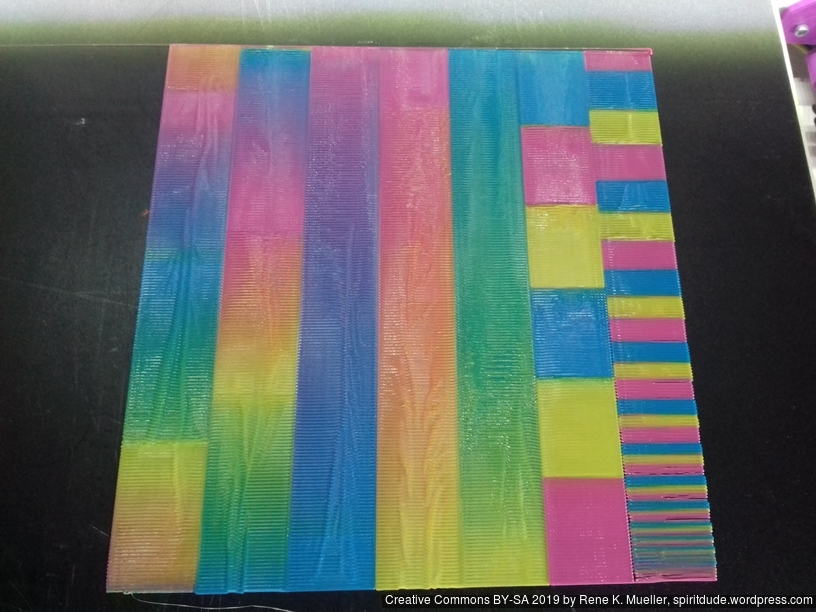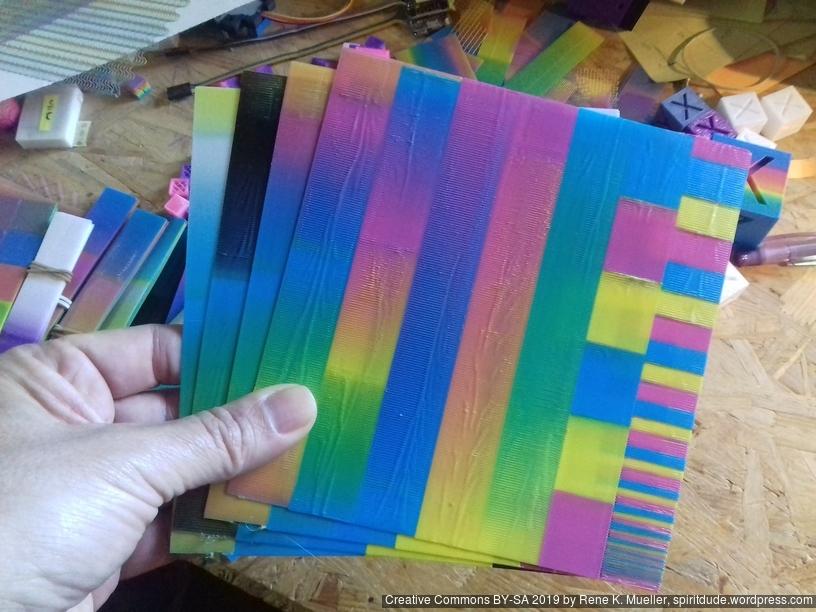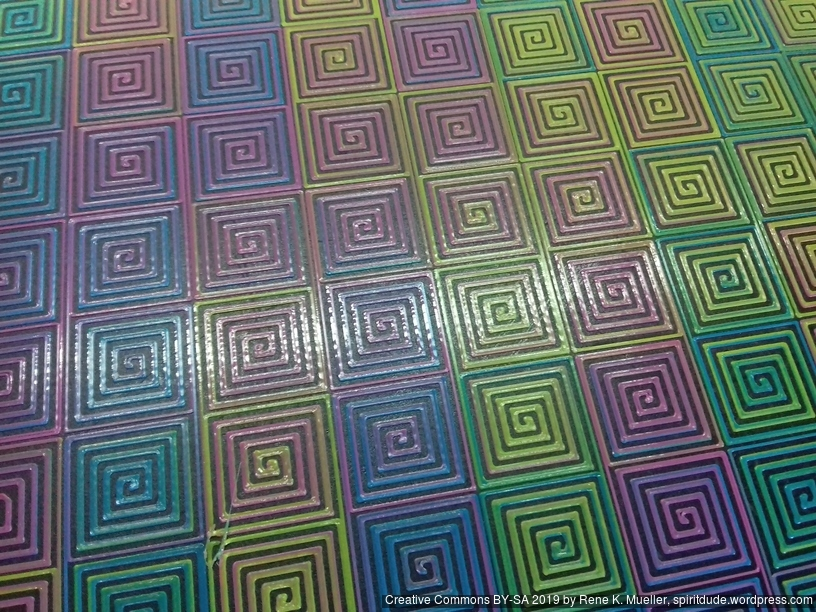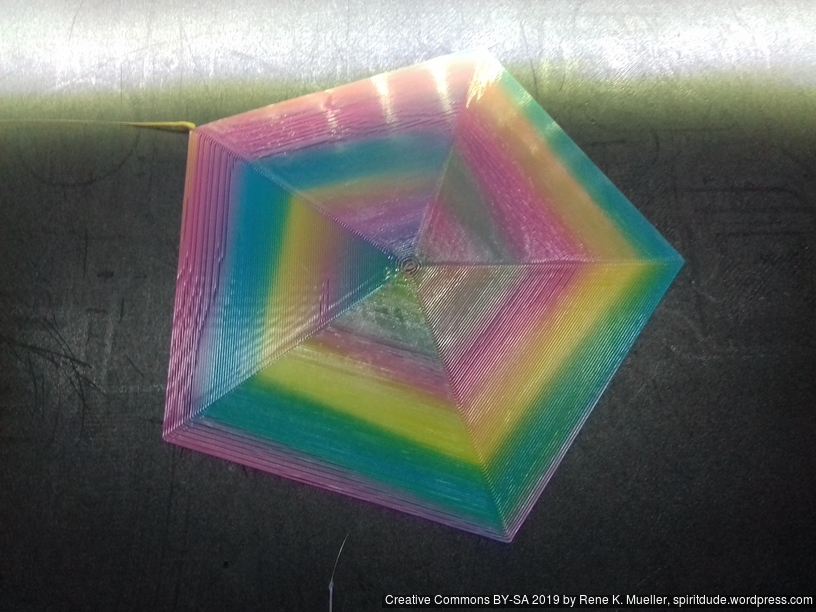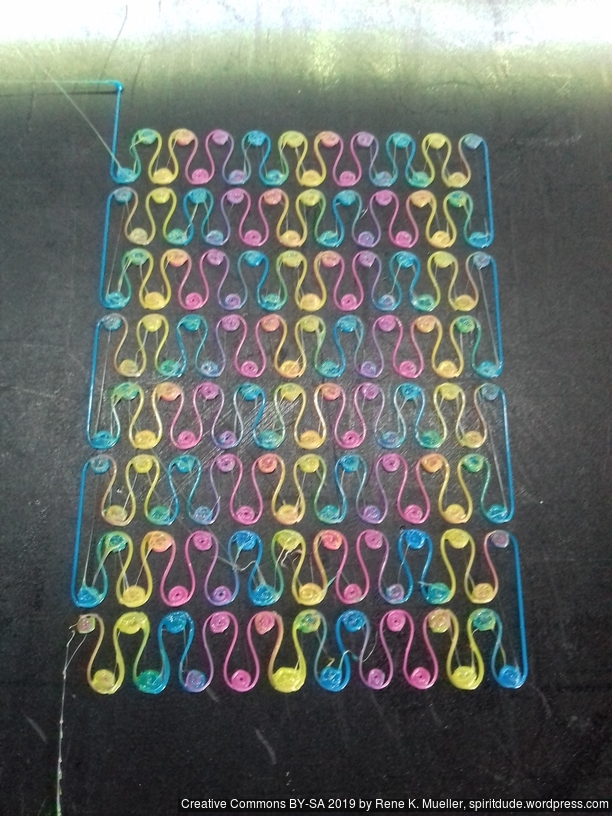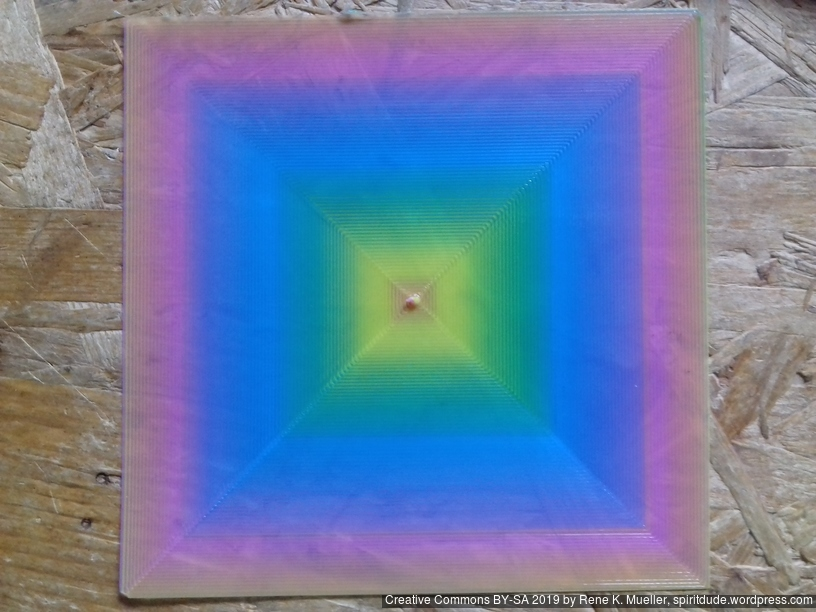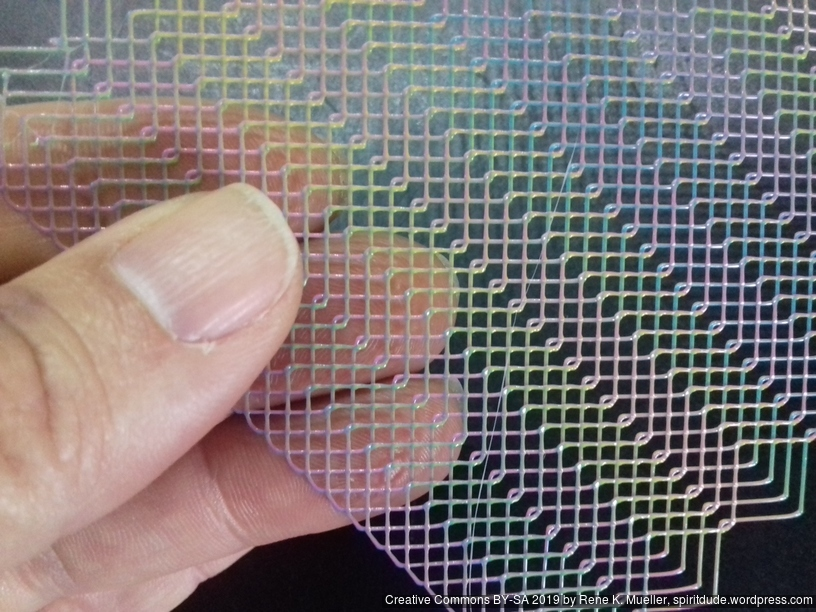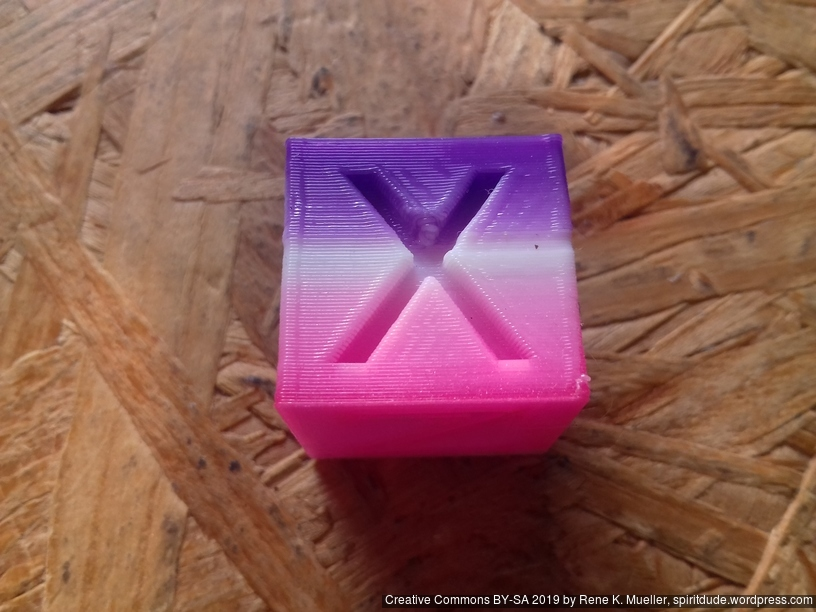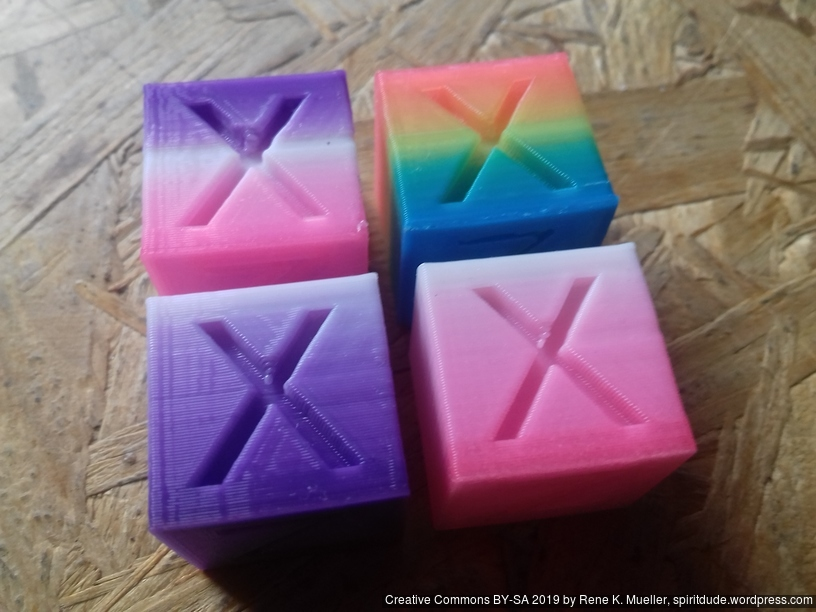That’s it.